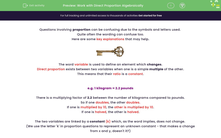# Work with Direct Proportion Algebraically

In this worksheet, students will find the values of unknown variables which are directly proportional to each other, using appropriate notation to express proportional relationships algebraically.Key stage:  KS 4

Year:  GCSE

GCSE Subjects:   Maths

GCSE Boards:   AQA, Eduqas, Pearson Edexcel, OCR,

Curriculum topic:   Ratio, Proportion and Rates of Change

Curriculum subtopic:   Ratio, Proportion and Rates of Change Direct and Inverse Proportion

Difficulty level:#### Worksheet Overview

Questions involving proportion can be confusing due to the symbols and letters used.

Quite often the wording can confuse too.

Here are some key explanations that may help.The word variable is used to define an element which changes.

Direct proportion exists between two variables when one is a simple multiple of the other.

This means that their ratio is a constant.

e.g. 1 kilogram = 2.2 pounds

There is a multiplying factor of 2.2 between the number of kilograms compared to pounds.

So if one doubles, the other doubles.

If one is multiplied by 10, the other is multiplied by 10.

If one is halved, the other is halved.

The two variables are linked by a constant (k) which, as the word implies, does not change.

(We use the letter 'k' in proportion questions to represent an unknown constant - that makes a change from x and y, doesn't it?)

We need to find the value of k each time, then substitute this into our formula to solve any problem involving these variables.

The symbol for direct proportion is:

So to write 'pay is directly proportional to time' in this way we would write:

Pay ∝ Time

This is where the letter 'k' can help us too.

If we want to express 'Pay' in relation to 'Time', we could say that:

Pay = k × Time

Let's look at these notations in action now using an example.

e.g. T is directly proportional to M. If T = 20 when M = 4, find:

a) T when M = 3

T ∝ M and T = k × M

Now let's substitute in what we know:

20 = k × 4

So to find k, we need to divide 20 by 4:

20 ÷ 4 = 5

Therefore, our multiplier (k) is 5.

Now we can substitute this multiplier into our expression, to find T when M = 3:

T = k × 3

T = 5 × 3

So T = 15 when M = 3.

b) M when T = 10

We can use the same expressions from above and the same multiplier for k:

T = k × M

10 = 5 × M

M = 10 ÷ 5 = 2

Okay - let's dive in!In this activity, you will find the values of unknown variables which are directly proportional to each other, using appropriate notation to express proportional relationships algebraically.

### What is EdPlace?

We're your National Curriculum aligned online education content provider helping each child succeed in English, maths and science from year 1 to GCSE. With an EdPlace account you’ll be able to track and measure progress, helping each child achieve their best. We build confidence and attainment by personalising each child’s learning at a level that suits them.

Get started